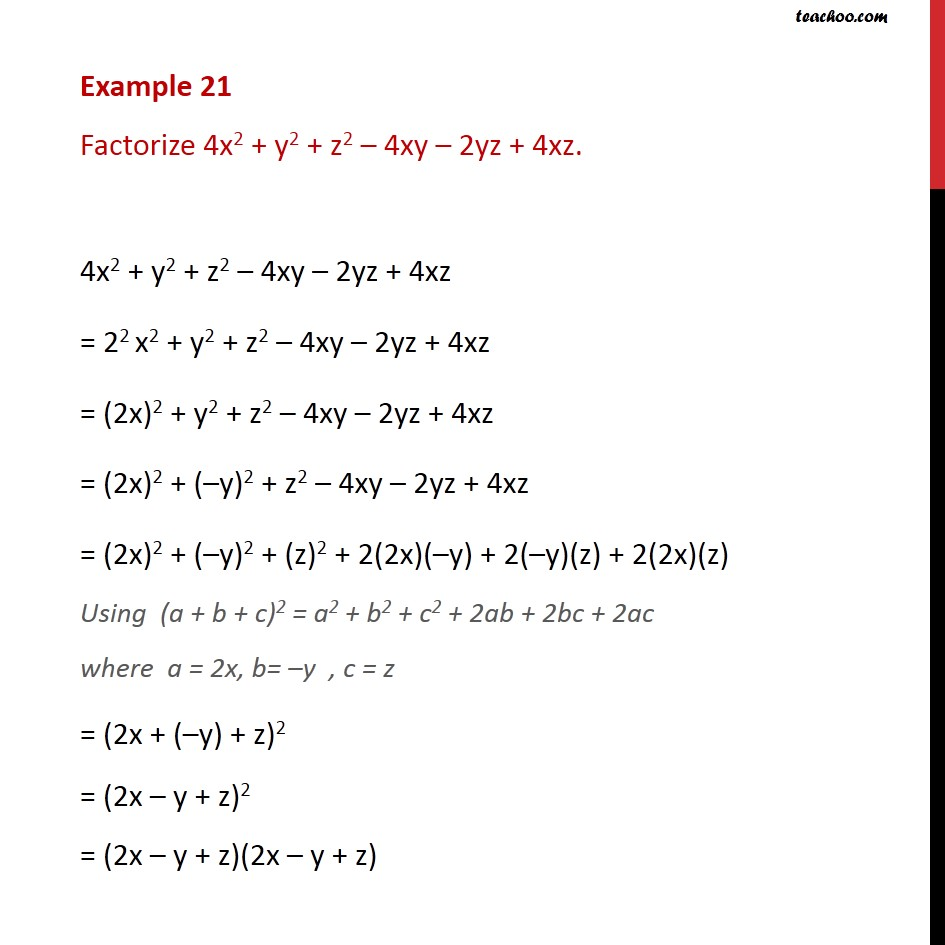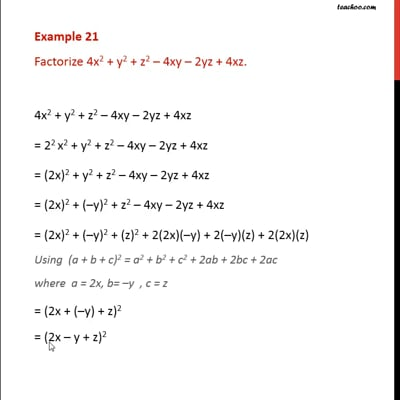Examples

Chapter 2 Class 9 Polynomials
Serial order wiseThis video is only available for Teachoo black users

Get live Maths 1-on-1 Classs - Class 6 to 12

### Transcript

Example 21 Factorize 4x2 + y2 + z2 – 4xy – 2yz + 4xz. 4x2 + y2 + z2 – 4xy – 2yz + 4xz = 22 x2 + y2 + z2 – 4xy – 2yz + 4xz = (2x)2 + y2 + z2 – 4xy – 2yz + 4xz = (2x)2 + (–y)2 + z2 – 4xy – 2yz + 4xz = (2x)2 + (–y)2 + (z)2 + 2(2x)(–y) + 2(–y)(z) + 2(2x)(z) Using (a + b + c)2 = a2 + b2 + c2 + 2ab + 2bc + 2ac where a = 2x, b= –y , c = z = (2x + (–y) + z)2 = (2x – y + z)2 = (2x – y + z)(2x – y + z)